GeeksforGeeks App
Open AppBrowser
Continue

# PostgreSQL – Random Number Generation

PostgreSQL provides the random() function that returns a random number between 0 and 1. In this article, we will look into the process of developing a user-defined function for generating random numbers within a range.

```Syntax:SELECT random();

```

If you try the above syntax it will lead to the following: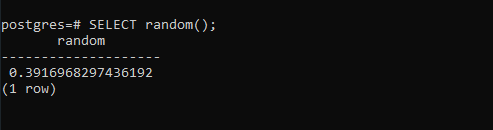To generate a random number between 1 and 10, you use the following statement:

```SELECT random() * 10 + 1 AS RAND_1_10;

```

If you try the above syntax it will lead to the following: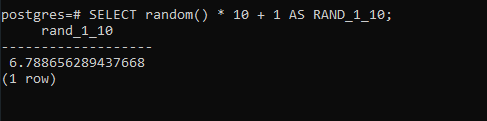If you want to generate the random number as an integer, you apply the floor() function to the expression as follows:

```SELECT floor(random() * 10 + 1)::int;

```

The above query results in the following: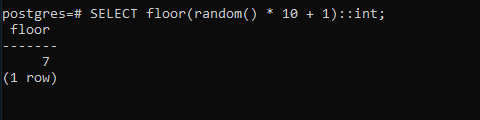Generally, to generate a random number between two integers l and h, you use the following statement:

```SELECT floor(random() * (h-l+1) + l)::int;

```

To create a user-generated function that returns a random number between two numbers l and h:

```CREATE OR REPLACE FUNCTION random_between(low INT ,high INT)
RETURNS INT AS
\$\$
BEGIN
RETURN floor(random()* (high-low + 1) + low);
END;
\$\$ language 'plpgsql' STRICT;

```

The following statement calls the random_between() function and returns a random number between 1 and 100:

```SELECT random_between(1,100);

```

Output: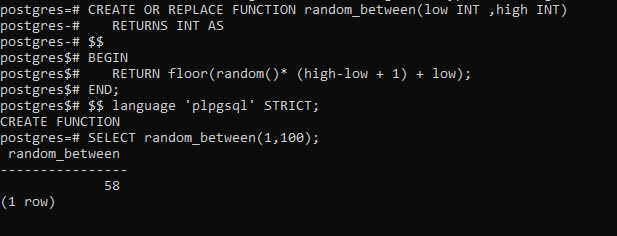If you want to get multiple random numbers between two integers, you use the following statement:

```SELECT random_between(1,100)
FROM generate_series(1,5);

```

Output: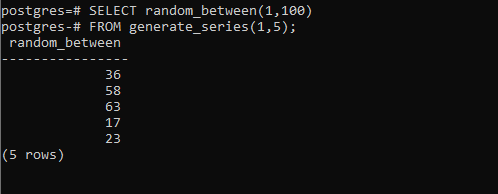My Personal Notes arrow_drop_up